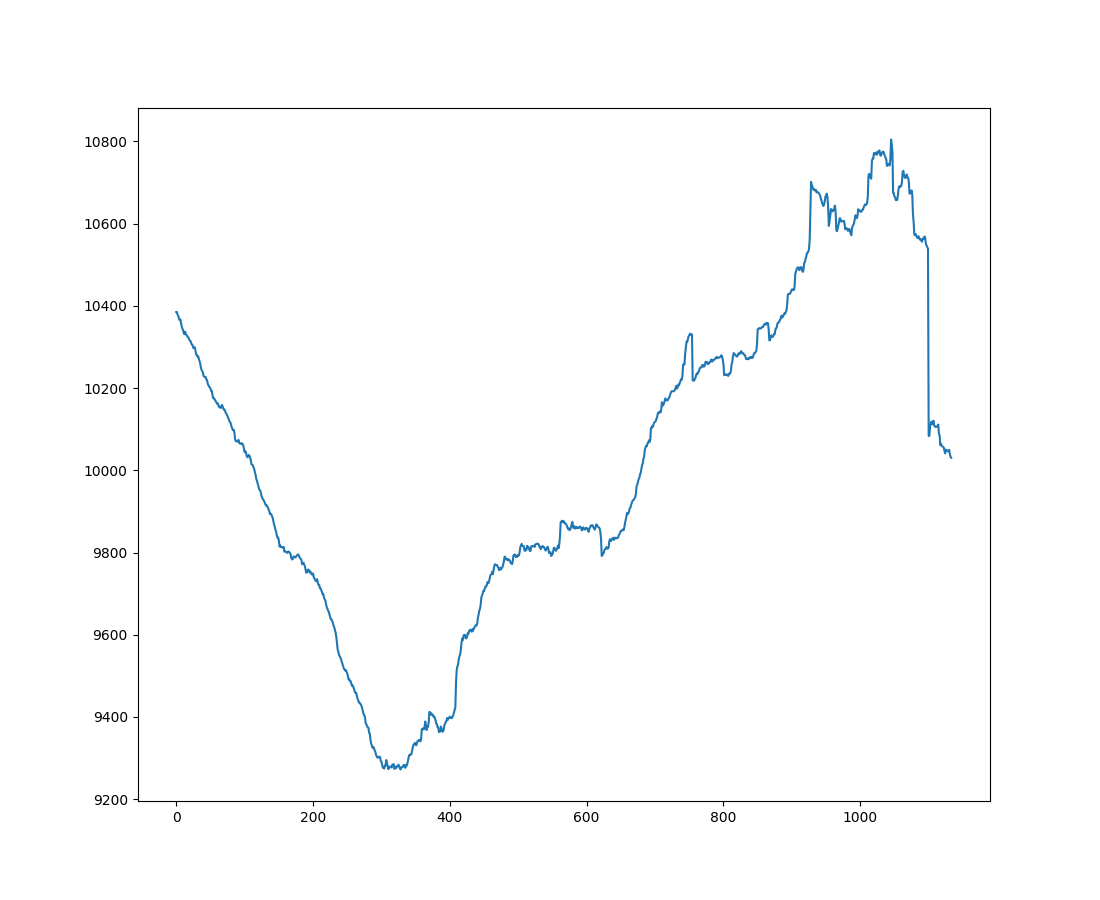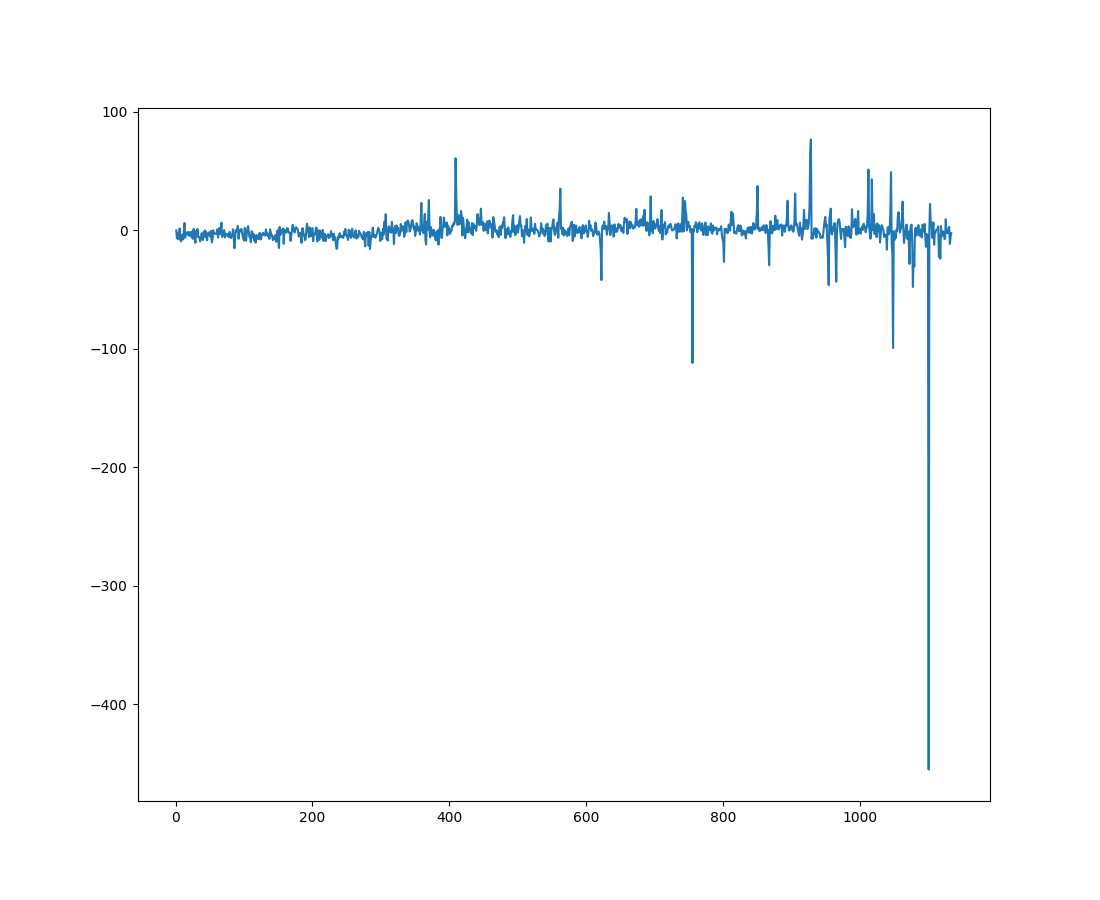Course Content

# Data Preprocessing

2. Processing Quantitative Data

3. Processing Categorical Data

4. Time Series Data Processing

Data Preprocessing

##Stationarity

One of the main steps is the process of converting a non-stationary time series into a stationary one by eliminating the trend, seasonality, and other factors that affect the change in the statistical properties of the series over time. A transformed stationary time series can be more predictable and easier to analyze than a non-stationary series. There are various methods of transforming data to stationary:

### Differencing

Differencing - calculating the difference between the time series's current and previous value of the time series. But how to choose the order of differencing? If the first differences fail to revolve around a constant mean and variance, then we find the second differencing using the values of the first differencing. You can repeat this until you get a stationary series.

You can also plot the differenced series and check to see if there is a constant mean and variance to determine whether or not the series is sufficiently differenced.

### Decomposition

Decomposition - breaking down the time series into its trend, seasonality, and random noise components.

### Box-Cox transformation

Box-Cox transformation is a method that generalizes the natural logarithm transform and converts non-normal data to more normal distribution.

### Outlier removal

Outlier removal - a method that removes outliers from the non-stationary time series, which helps improve its stationarity.

In the example below, we will consider how to implement the transformation of data into stationary data using the decomposition method:You can look at the plots below. The first - is the original dataset, and the second - is after the differencing method has been applied.